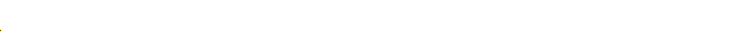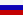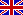Main
Extracting signal masked by the chaotic signal of electronic oscillator with delayed feedback

The discovery of the phenomenon of synchronization in chaotic dynamical systems has given rise to active development of secure communication methods using chaotic signal as a carrier. However, many chaotic communication schemes are not as secure as expected. A hidden message can be successfully unmasked even in communication schemes using masking chaotic signals of time-delay systems in spite of very high dimension and large number of positive Lyapunov exponents of chaotic attractors of time-delay systems.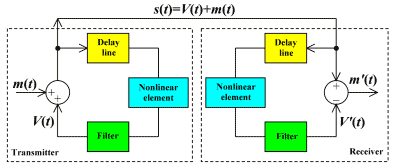A block diagram of a chaotic communication system based on a time-delayed feedback system is shown in the figure. For the case when the filter is a low-frequency first-order RC-filter the transmitter is described in the absence of information signal (m(t)=0) by the delay-differential equation RCV'(t)=-V(t)+ƒ(V(t0)), where V(t) and V(t0) are the delay line input and output voltages, respectively, τ0 is the delay time, R and C are the resistance and capacitance of the filter elements. The harmonic signal m(t) is nonlinearly mixed with the chaotic signal V(t). The signal s(t)=V(t)+s(t) is transmitted into the communication channel and simultaneously injected into the feedback circuit of the transmitter. In the experimental setup we recorded the signals m(t) and s(t) using an analog-to-digital converter. Parts of the time series and power spectra of these signals are shown in the figure by red and blue colors, respectively.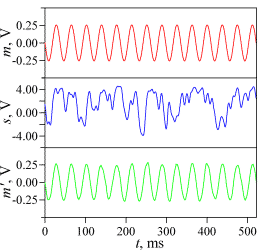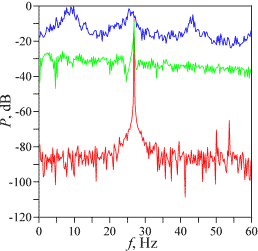A hidden message can be extracted from the time series of the transmitted signal s(t) even in the case when the transmitter parameters a priori unknown. To recover the nonlinear function ƒ and the parameters τ0 and RC of the transmitter we use the proposed by us method of time-delay system reconstruction from time series. Knowing the transmitter parameters one can construct the receiver and extract the message signal m′(t). Part of the time series of the extracted harmonic signal m′(t) and its power spectrum are shown in the figure by green color.

The method provides high accuracy of the transmitter parameters recovery and message extraction for the information signal amplitudes up to 10% of the carrier amplitude. However, the level of information signal in the considered communication scheme must be significantly lower, otherwise the chaotic signal will not provide enough masking.

The more detailed description of methods for encryption and decryption of information in communication schemes using masking chaotic signals of time-delay systems is given in the paper Ponomarenko V.I., Prokhorov M.D., Phys. Rev. E, 2002, V.66, 026215.